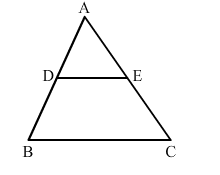# In ∆ABC, D and E are the midpoints of AB and AC, respectively.

Question:

In ∆ABCD and E are the midpoints of AB and AC, respectively. Find the ratio of the areas of ∆ADE and ∆ABC.Solution:

It is given that D and E are midpoints of AB and AC.
Applying midpoint theorem, we can conclude that DE ">BC.
Hence, by B.P.T., we get:

$\frac{A D}{A B}=\frac{A E}{A C}$

Also, $\angle A=\angle A$

Applying SAS similarity theorem, we can conclude that $\triangle A D E \sim \triangle A B C$.

Therefore, the ratio of the areas of these triangles will be equal to the ratio of squares of their corresponding sides.

$\therefore \frac{\operatorname{ar}(\Delta A D E)}{\operatorname{ar}(\Delta A B C)}=\frac{D E^{2}}{B C^{2}}$

$=\frac{\left(\frac{1}{2} B C\right)^{2}}{B C^{2}}$

$=\frac{1}{4}$